# Higher derivative test need not be conclusive for infinitely differentiable function at isolated critical point

This article describes a situation, or broad range of situations, where a particular test or criterion is inconclusive, i.e., it does not work as intended to help us determine what we would like to determine. This is either because one of the hypotheses for the test fails or we land up in an inconclusive branch of the test.
The test is higher derivative test. See more inconclusive cases for higher derivative test | conclusive cases for higher derivative test

## Statement

Suppose we are given a function$f$ defined on$\R$ and choose a point$c$ such that:

•$f$ is infinitely differentiable at$c$ (and in fact, we can choose$f$ to be infinitely differentiable everywhere).
•$f$ has a critical point at$c$, i.e.,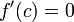$f'(c) = 0$, and this is an isolated critical point, i.e., there is no critical point to the immediate left or immediate right of$c$ (if we wish, we can choose things so that$c$ is the only critical point for$f$).
• For all positive integers$k$,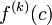$f^{(k)}(c)$, i.e., the higher derivative of$f$ at$c$, takes the value zero. In other words,$f'(c) = 0, f''(c) = 0, \dots$.

Then, the higher derivative test fails for$f$. In particular, any of these is possible for$f$ at$c$: strict local maximum, strict local minimum, point of increase (max from left, min from right), point of decrease (min from left, max from right).

Note that because of the isolated critical point assumption,$f$ cannot be constant on the immediate left or immediate right of$c$.

## Proof

### One-sided examples

For convenience, we take$c = 0$ in the examples below.

What we want Function description at point and on appropriate side (we ignore the other side)
strict local maximum from left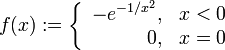$f(x) := \left\lbrace \begin{array}{rl} -e^{-1/x^2}, & x < 0 \\ 0, & x = 0 \\\end{array} \right.$
strict local minimum from left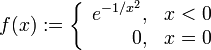$f(x) := \left\lbrace \begin{array}{rl} e^{-1/x^2}, & x < 0 \\ 0, & x = 0 \\\end{array} \right.$
strict local maximum from right$f(x) := \left\lbrace \begin{array}{rl} -e^{-1/x^2}, & x > 0 \\ 0, & x = 0 \\\end{array} \right.$
strict local minimum from right$f(x) := \left\lbrace \begin{array}{rl} e^{-1/x^2}, & x > 0 \\ 0, & x = 0 \\\end{array} \right.$

### Two-sided examples

For convenience, we take$c = 0$ in the examples below.

What we want Function description at point and on left and right
strict local maximum (two-sided)$f(x) := \left\lbrace \begin{array}{rl} -e^{-1/x^2}, & x \ne 0 \\ 0, & x = 0 \\\end{array} \right.$
strict local minimum (two-sided)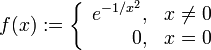$f(x) := \left\lbrace \begin{array}{rl} e^{-1/x^2}, & x \ne 0 \\ 0, & x = 0 \\\end{array} \right.$
point of increase for the function (max from left, min from right)$f(x) := \left\lbrace \begin{array}{rl} -e^{-1/x^2}, & x < 0 \\ e^{-1/x^2}, & x > 0 \\0, & x = 0 \\\end{array} \right.$
point of decrease for the function (min from left, max from right)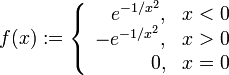$f(x) := \left\lbrace \begin{array}{rl} e^{-1/x^2}, & x < 0 \\ -e^{-1/x^2}, & x > 0 \\0, & x = 0 \\\end{array} \right.$